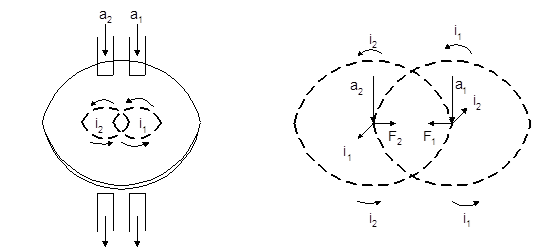### Theory of Torque Formation

Electromagnetic induction relays operate on the principle of induction motor and are widely used for protecting relaying purposes involving ac quantities. They are not used with dc quantities owing to the principle of operation. An induction relay essentially consists of a pivoted aluminium disc placed in two alternating magnetic fields of the same frequency but displaced in time and space. The torque is produced in the disc by the interaction of one of the magnetic fields with the currents induced in the disc by the other.

Figure 2.5 (i) shows the two ac fluxes Φ2 and Φ1 differing in phase by an angle α, induces an e.m.f.’s in disc and causes the circulation of eddy currents i2 and i1 respectively. These currents lag behind their respective fluxes by 90o.a1 = Φ1= Flux 1; a2 = Φ2 = Flux 2

Fig. 2.5 Elementary arrangement of Induction relay

Referring to figure 2.5 (ii) where the two ac fluxes and induced currents are shown separately for clarity, let

Φ1  = Φ1max sin ωt  & Φ2 = Φ2max sin (ωt + α)

Where, Φ1 and Φ2 are the instantaneous values of fluxes and Φ2 leads Φ1 by an angle α.

Voltages induced in the rotor are

e1     α       i1      and      e2     α       i2

Assuming that the paths in which the rotor currents flow have negligible self-inductance, the rotor currents will be in phase with their voltages.

i1      α       dΦ1 / dωt

α       d (Φ1max sin ωt) / dωt

α       Φ1max cos ωt

and   i2    α   dΦ2 / dωt

α d [Φ2max sin (ωt + α)] / dωt

α       Φ2max cos (ωt + α)

Now, the current produced by the flux interacts with the other flux and vice versa. The forces are

F1     α       Φ1 x  i2

and  F2     α       Φ2 x  i1

Figure 2.5 (ii) shows that the two forces are in opposition. Therefore, the net force F (or torque - T) at an instant considered is

F       α       F2     ­­­­ F1

α       Φ2 x  i1    Φ1 x  i2

α       Φ1max   x   Φ2max   x  sin α

α       Φ1   x   Φ2   x  sin α                                   …………….   (1)

Where, Φ1 & Φ2 are the r.m.s. values of the fluxes & the suffix ‘m’ is dropped and

sin α   =  sin (ωt + α - ωt)   =  [sin (ωt + α) cos ωt   - cos (ωt + α) sin ωt]

i.e. sin (A - B)         =       sin A  cos B – cos A  sin B

The following important conclusions can be drawn from the above expression (1) for torque:

1. Two alternating fluxes with a phase shift are needed for torque production, i.e. a single alternating flux would not produce torque.

2. Maximum torque is produced when two alternating flux are shifted in phase by 90o.

3. The resultant torque is steady as it is not a function of time as time ‘t’ is not involved in expression of torque.

4. The direction of net force and hence the direction of motion of the disc depends upon which flux is leading.